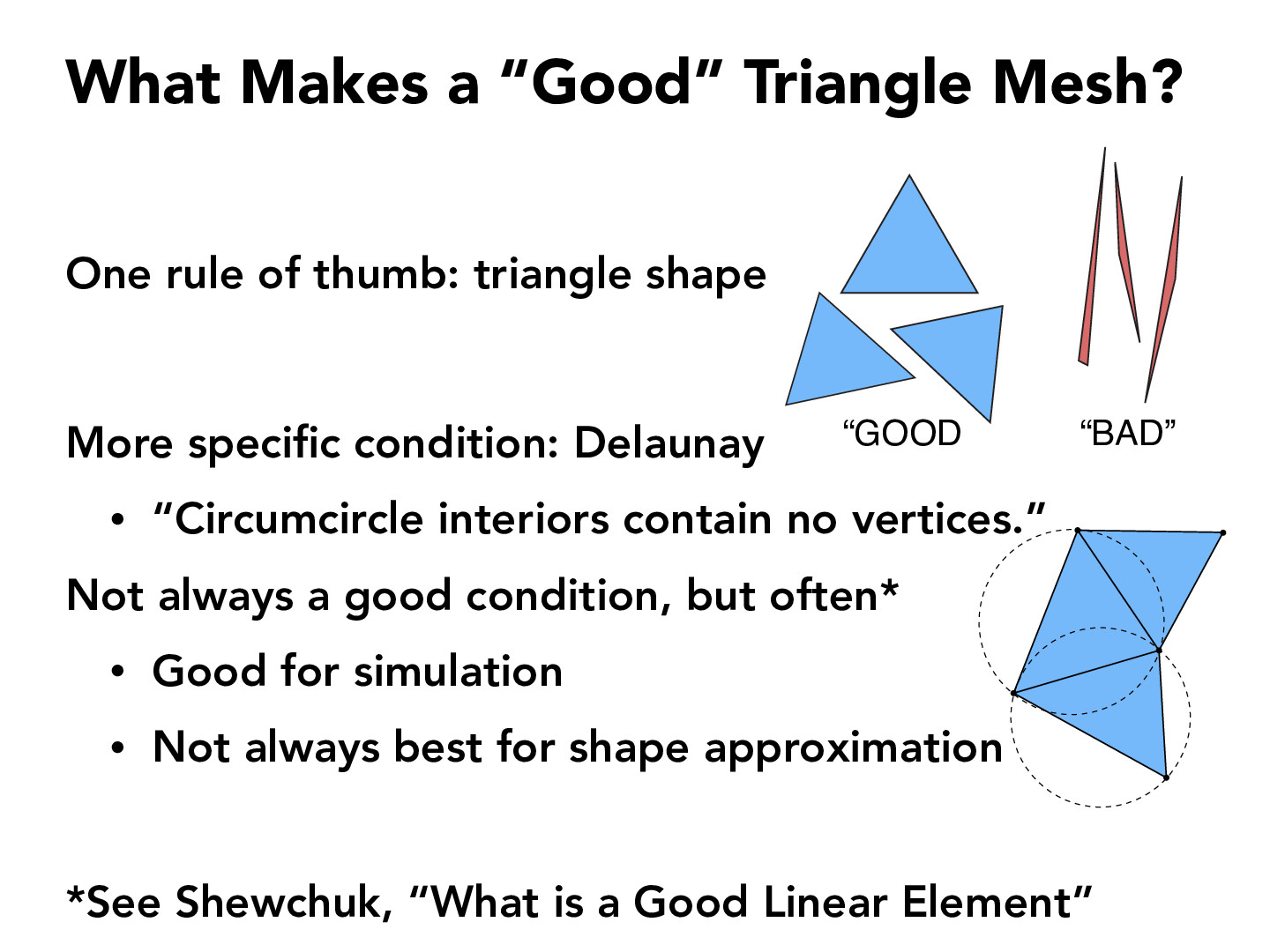You are viewing the course site for a past offering of this course. The current offering may be found here.Lecture 8: Meshes and Geometry Processing (73)susan-lin

I read a little bit of Shewchuk's paper, which mentions the paper of Babuska and Aziz. This was quite interesting because (based on my understanding) while accuracy degrades when angles approach 180 degrees, smaller angles (granted the largest angle in the triangle doesn't become too large) aren't necessarily harmful to interpolation accuracy or discretization error (Figure 2 in the paper illustrates this well)SourMongoose

Using the Delaunay condition, it seems a good indicator of a "good" triangle would be the area of the triangle divided by the area of the circumcircle, as this ratio becomes disproportionately small for skinnier triangles.camrankolahdouz

I think I understand why the valence of two triangles meshes matter but I don't see why this Delaunay condition matters. For this class do we just take it as fact.huangwl18

The Delaunay triangulation can also be interpreted as maximizing the smallest angles in the triangles created. By interpreting this way, we can frame this as an optimization problem in which we maximize the sum of squared of all the angles (the best case is where all angles are 60 degrees). Is this interpretation correct?

You must be enrolled in the course to comment